# Symmetry in Chaos

Written by Paul Bourke
March 2023

The following is based upon systems of equations found in "Symmetry in Chaos, A Search for Pattern in Mathematics, Art, and Nature" (the second edition) by Michael Field and Martin Golubitsky. They ask the question whether there are aperiodic chaotic attractors that generate symmetric patterns? Can there be order within chaotic systems?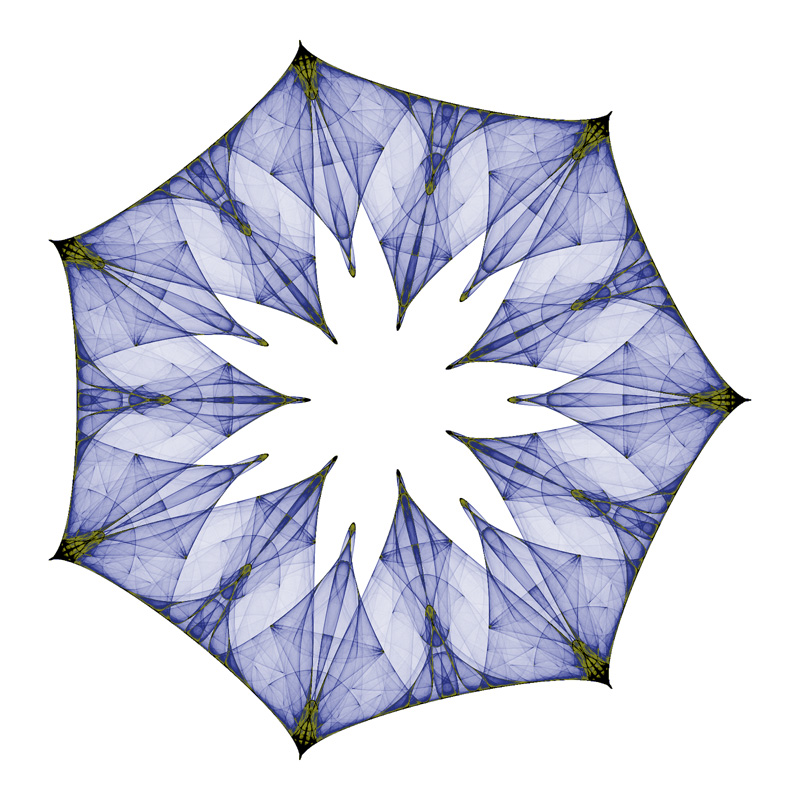Shield

The attractors are based upon the following formula for the m+1'th term in the series given 5 parameters a0 a1 ... a4. The integer n is the degree of symmetry. The z is complex valued and all an are real valued.Many of the examples presented here are named as published in the book and by other experimenters. The parameters a0 a1 ... a4 for some are provided in the book and subsequent papers, where they are denoted as lambda, alpha, beta, omega and gamma respectively.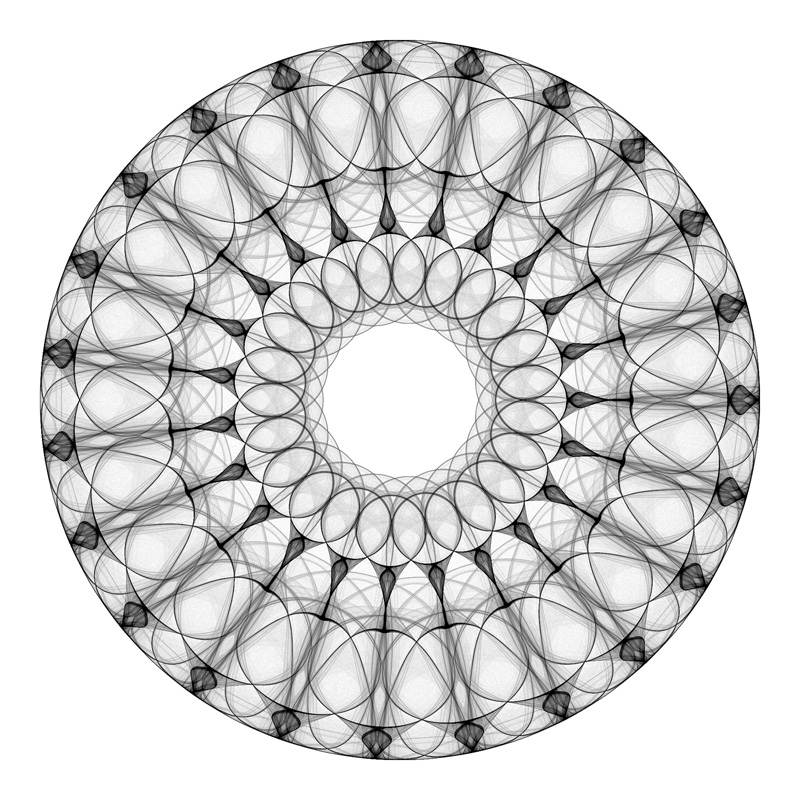Kachina Dolls

The images here are generated by iterating the series typically 1,000 million times. This is performed in two passes, the first pass with many fewer iterations is used to find the bounds of the attractor on the complex plane, the second pass actually "draws" the attractor points. The process of drawing involves treating the bounded region of the complex plane as a 2D histogram, each time the series passes through a pixel region on the plane the histogram at that location is incremented. One might imagine the 2D histogram as a height field, a larger values at a point indicate that the complex series passed through that pixel more often than a point with a smaller value. At the end of the process the histogram is mapped onto colours depending on the histogram values, there are many ways to do this based on aesthetic grounds.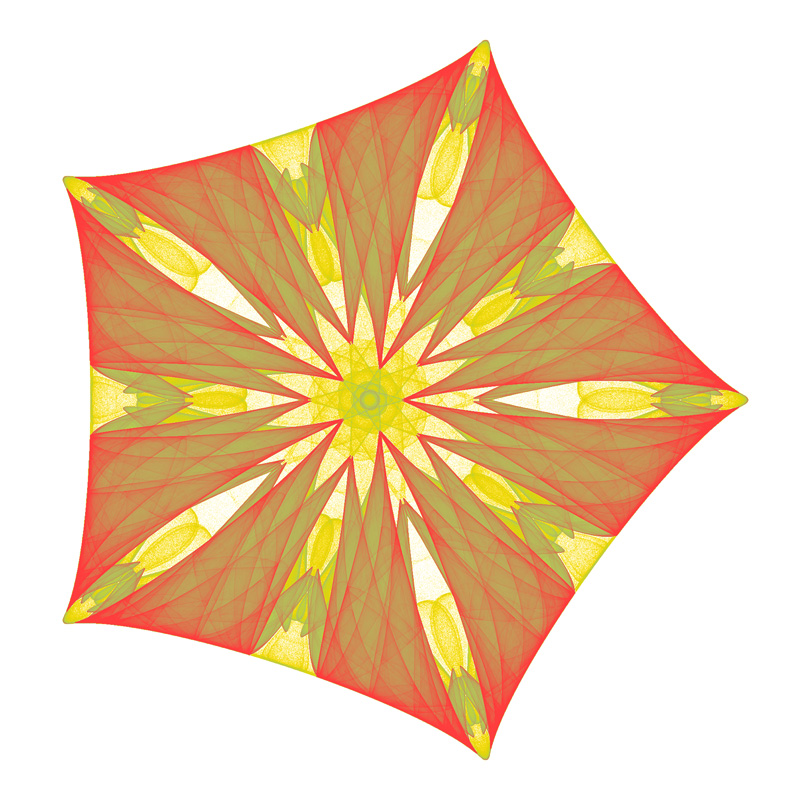Emperors Cloak

A rough guide to parameters.

• The value of n determines the rotational symmetry of the image, it should be greater than or equal to 2.

• a0 is usually between -3 and 3, values between -1 and 1 are generally less interesting.

• a1 should have the opposite sign as a0, and be in the same range.

• a2 perturbs the attractor, it can be 0.

• a3 controls bilateral symmetry, 0 will result in mirror symmetry. Otherwise typical values are between -1 and 1.

• a4 typically between -1 and 1 and not too close to zero.Santa Chiara

Unfortunately, for a system of equations with 6 parameters and therefore a large parameter space, the set of parameters that yield "interesting" results is quite small.Pentagonal attractorChaotic flowerSwirling StreamersTriangular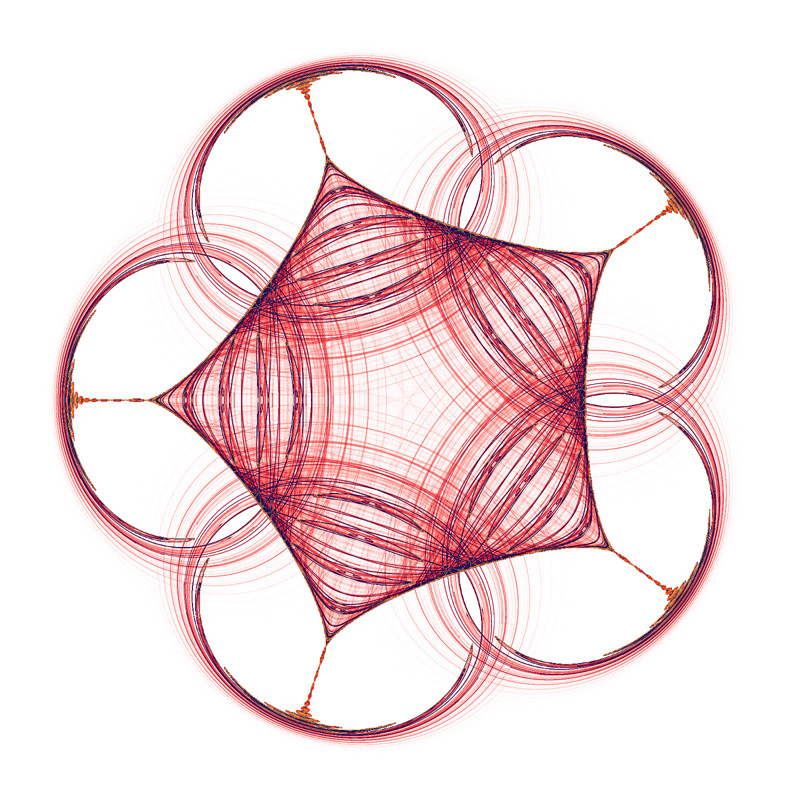Pentagonal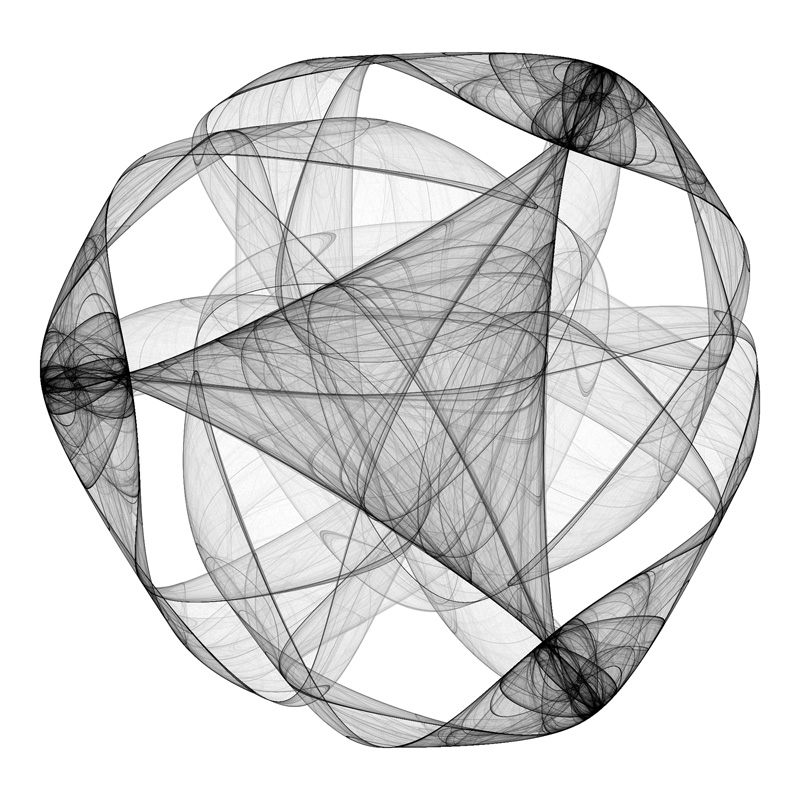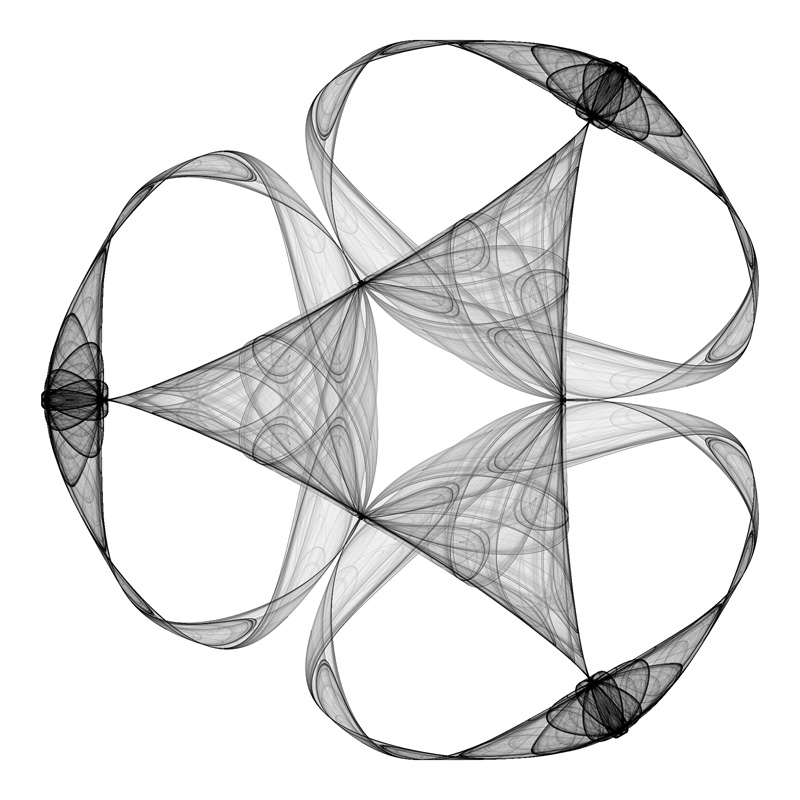Trinity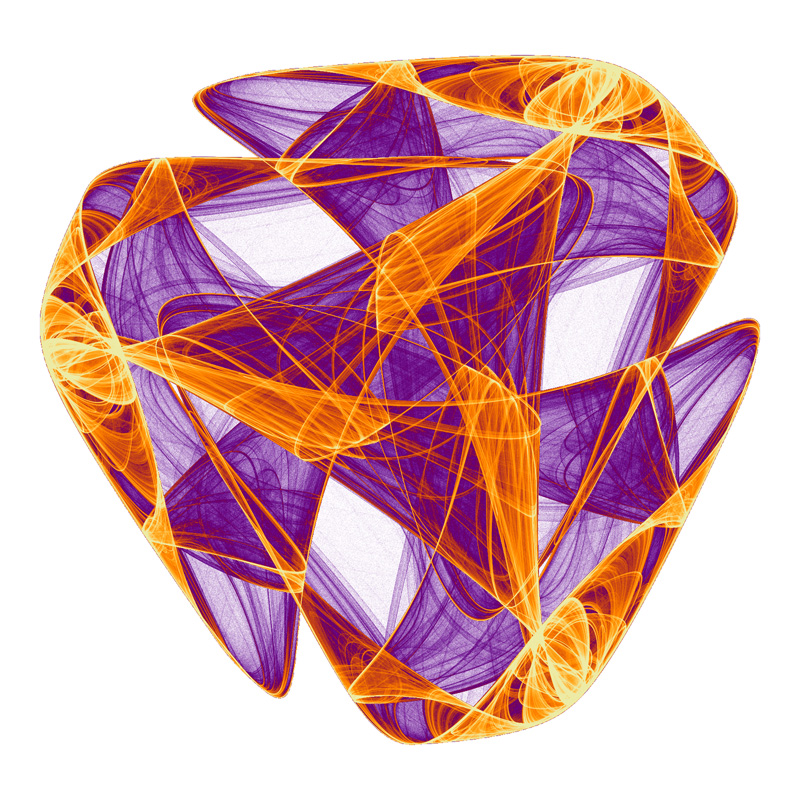Clam Triple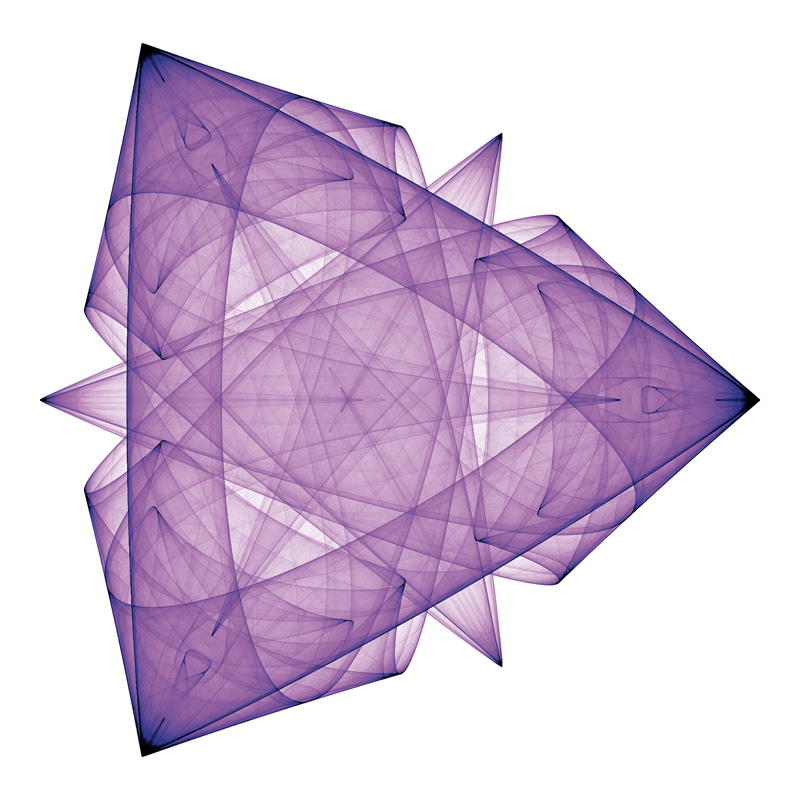Golden FlintstoneFrench Glass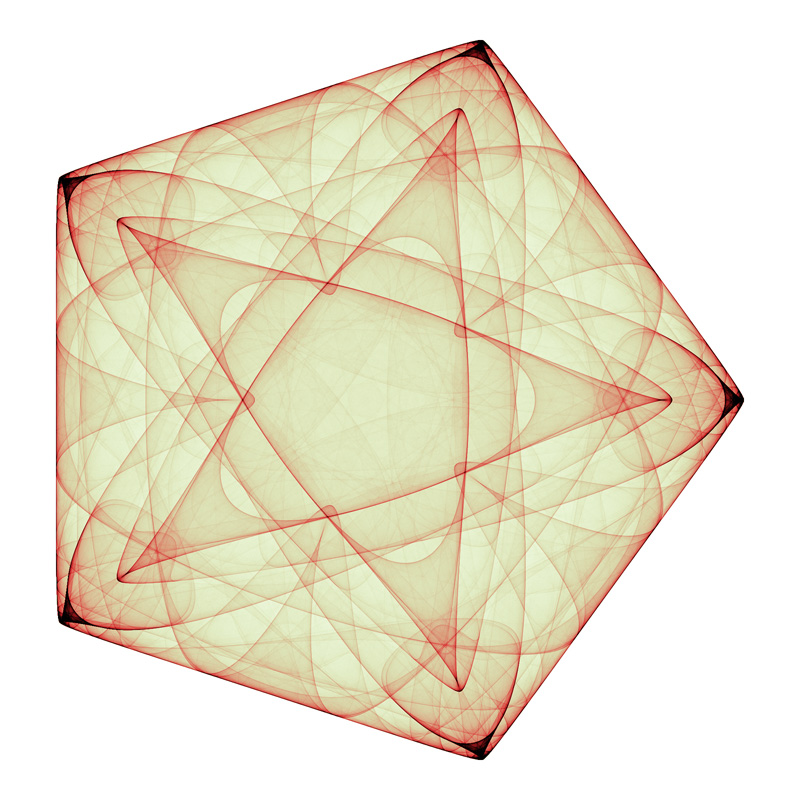Pentacle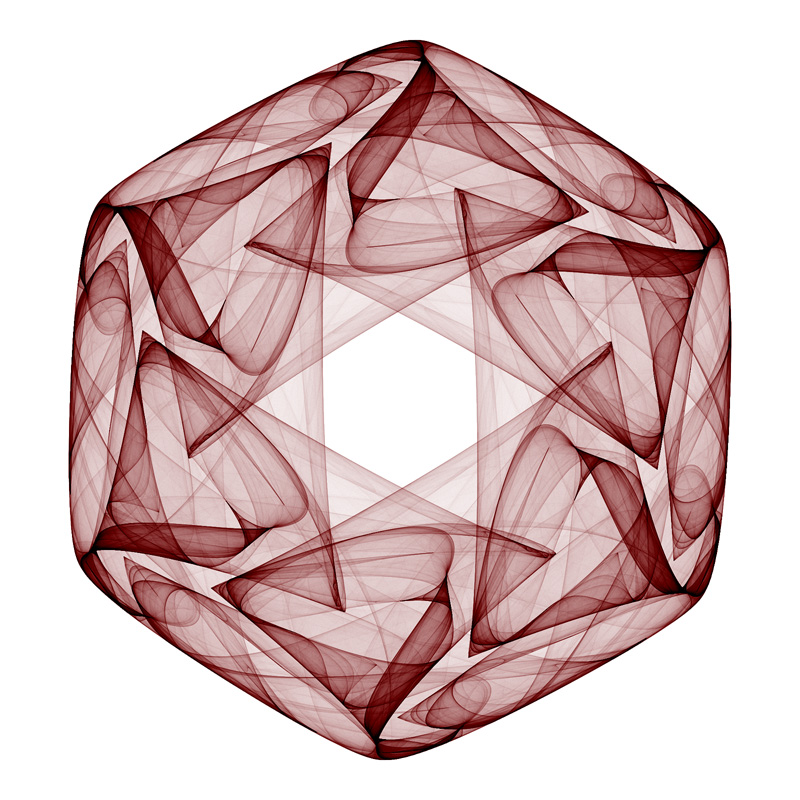Star of DavidHalloween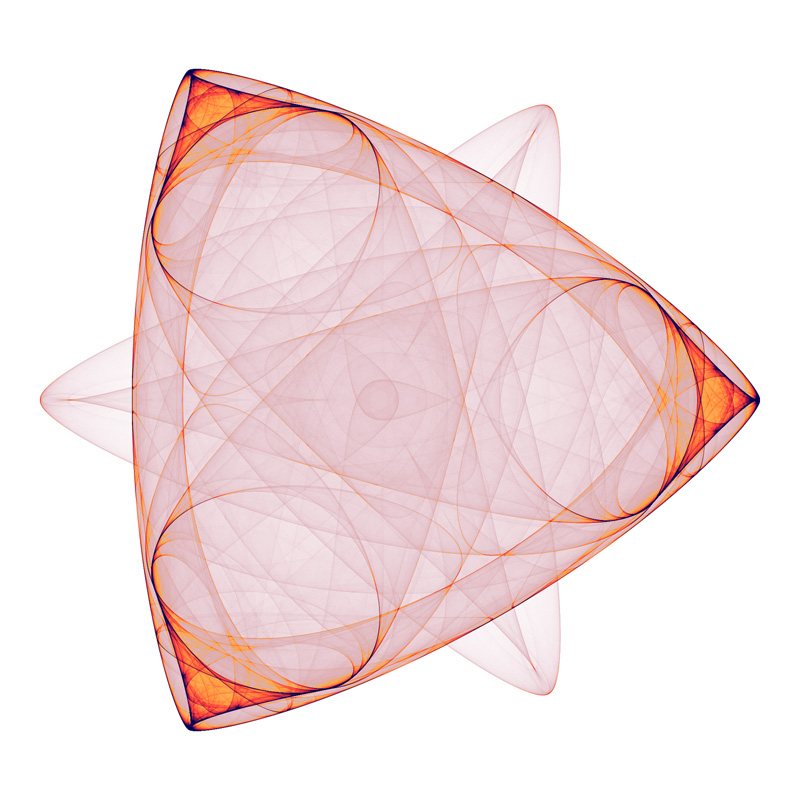Dual trianglesSquarePentiumPendulum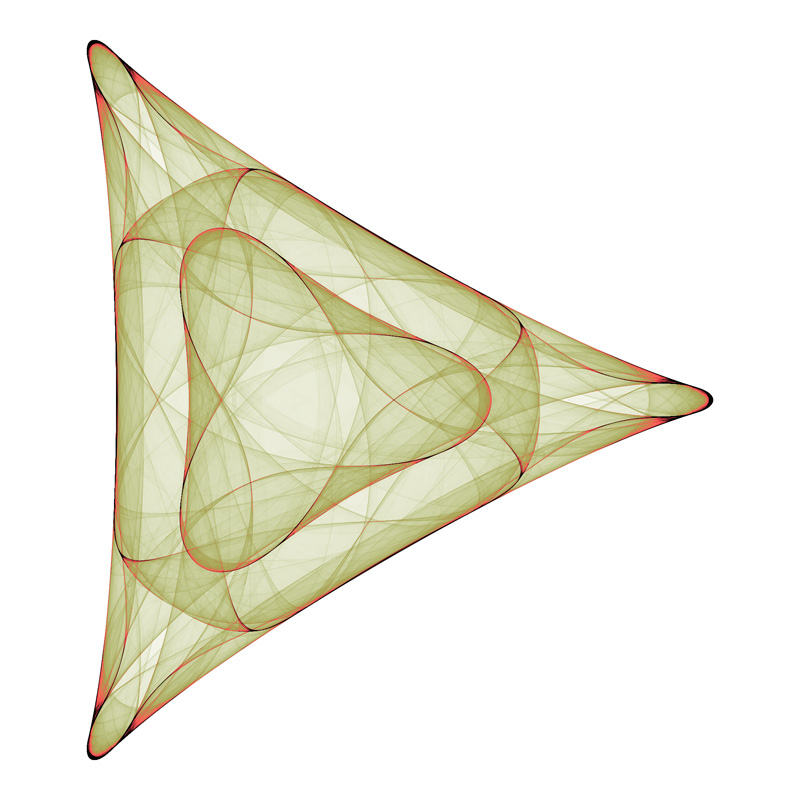The Trampoline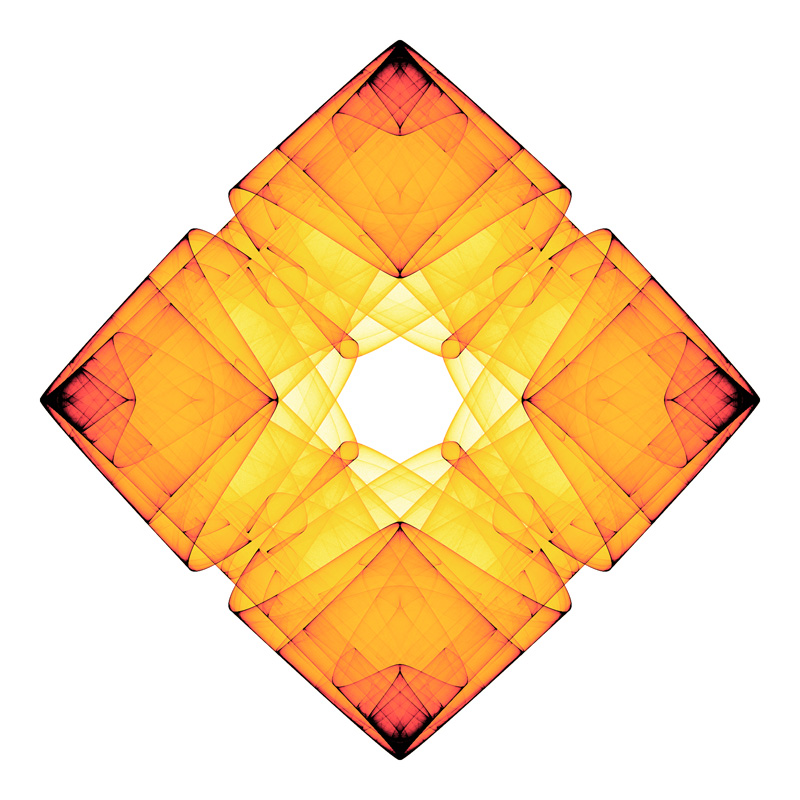Origami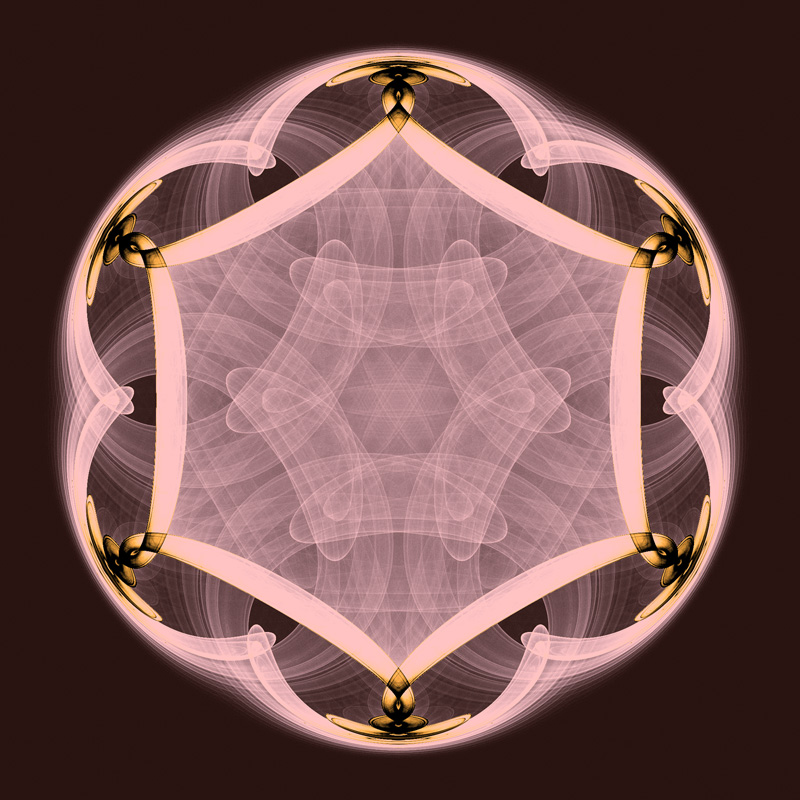Fuzzy Knot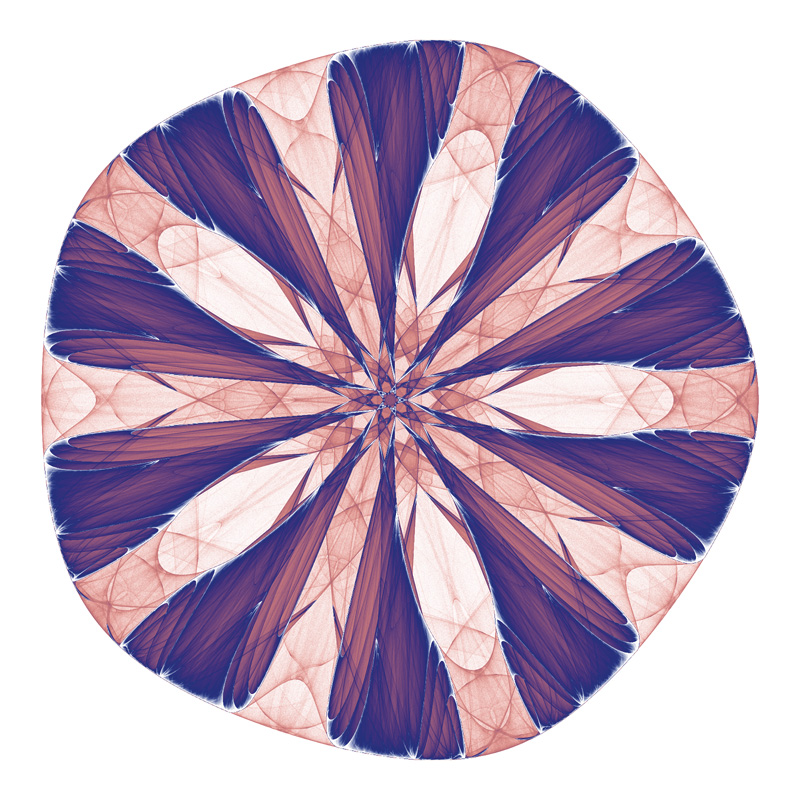Christmas Bow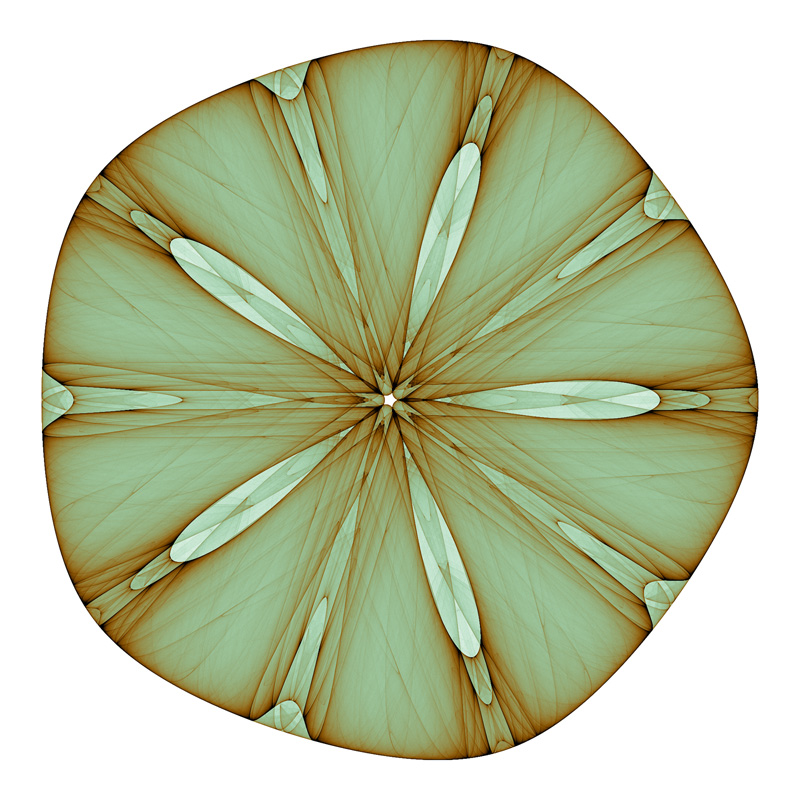Sand dollar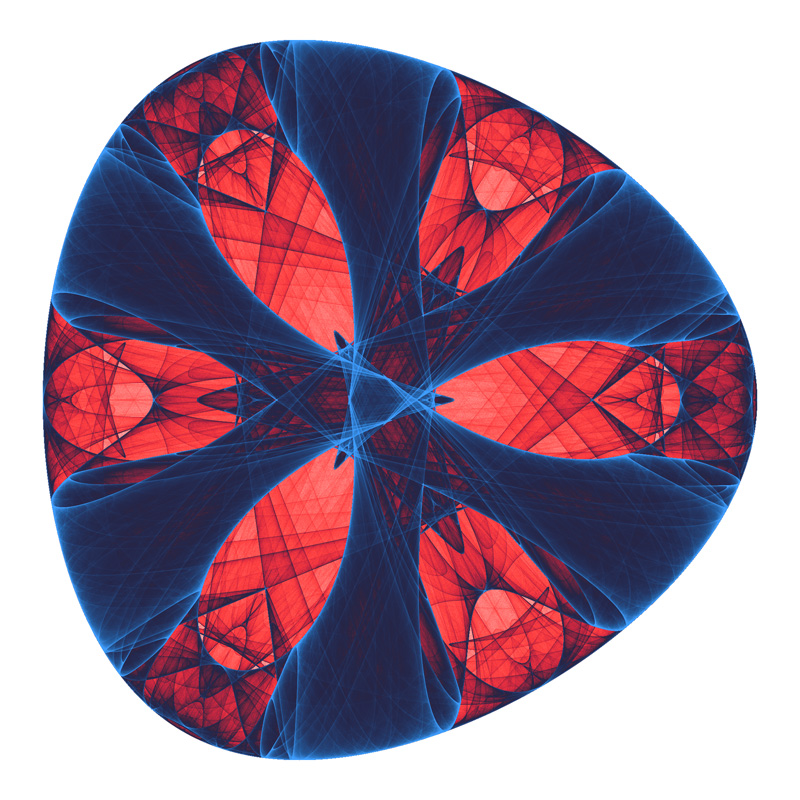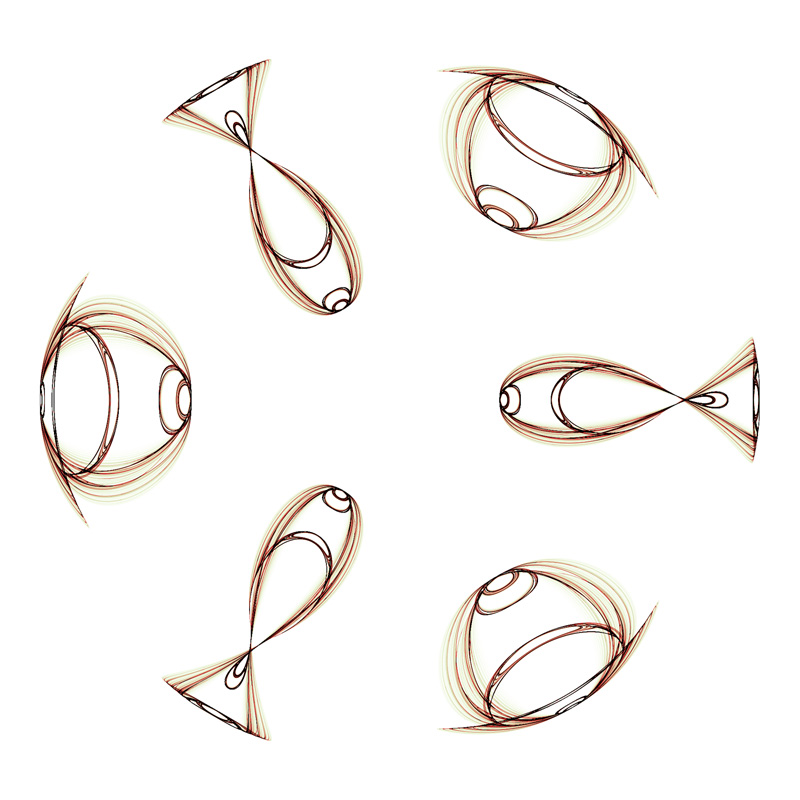Fish and eye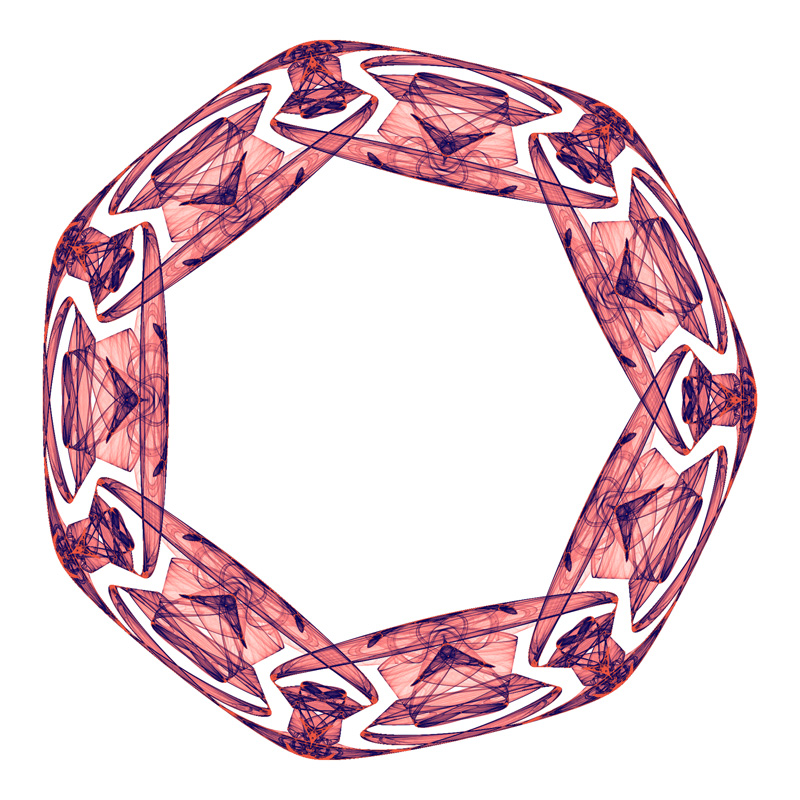Mayan bracklet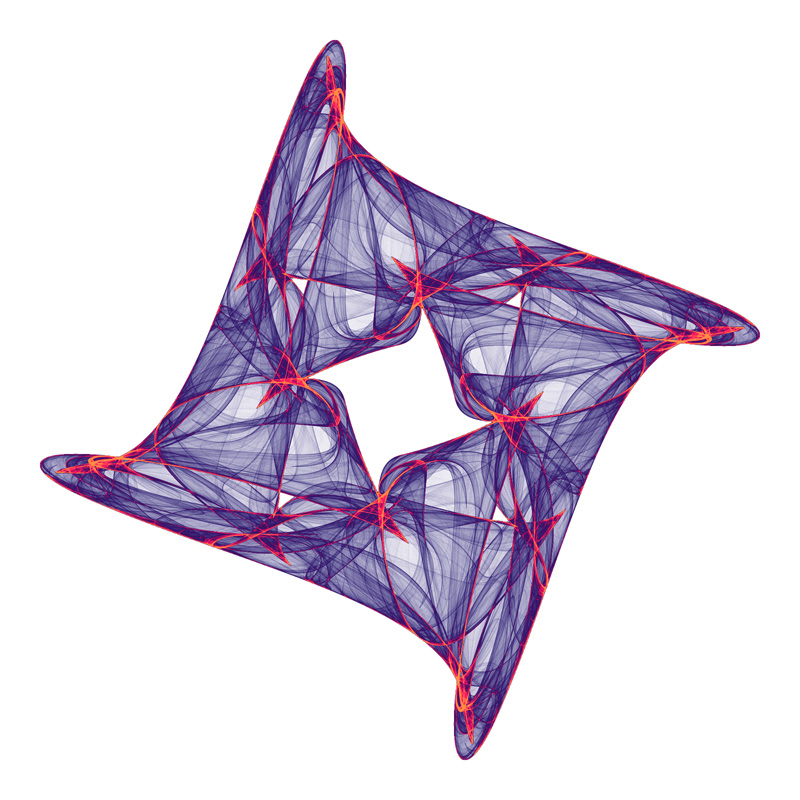Tangle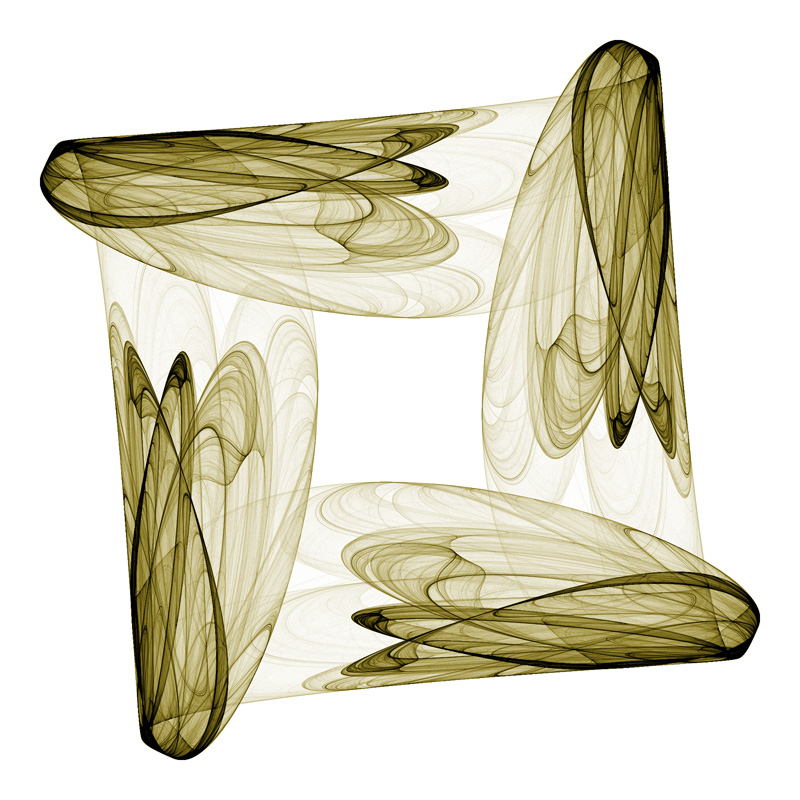Insect wings

A modified equation is also presented from the book as follows.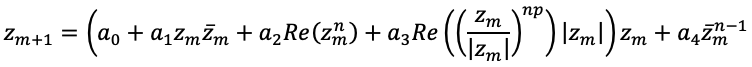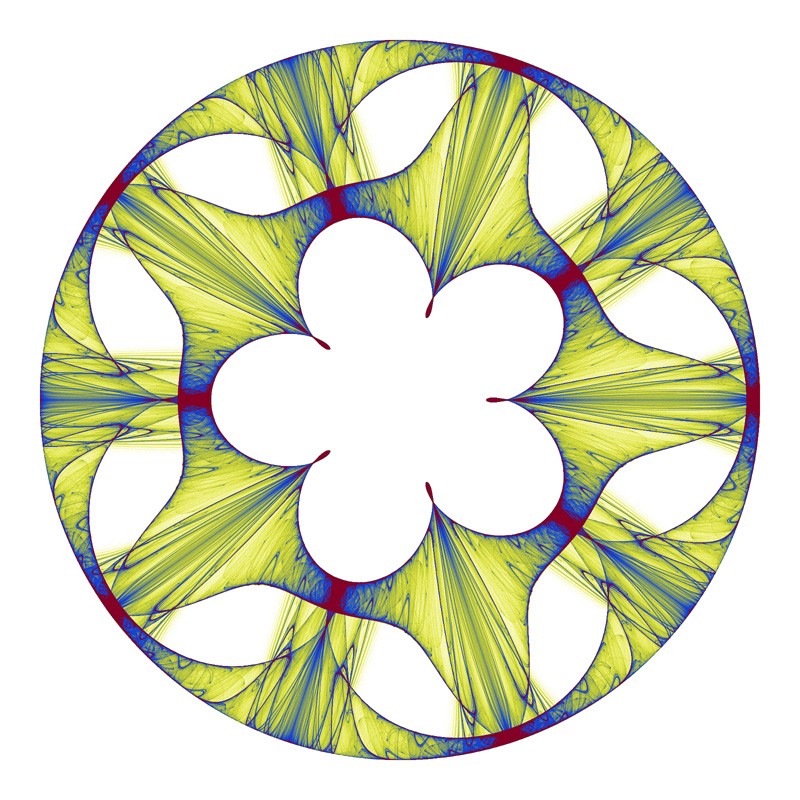Gothic MedallionMercedes-BenzSunflower

Random parameter search.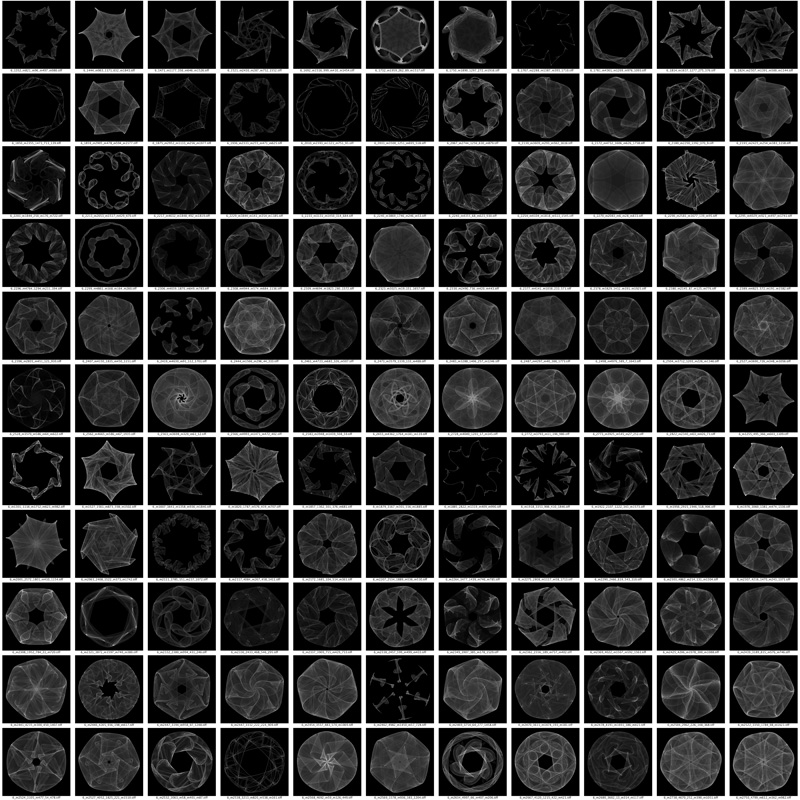6 fold symmetry3 fold symmetry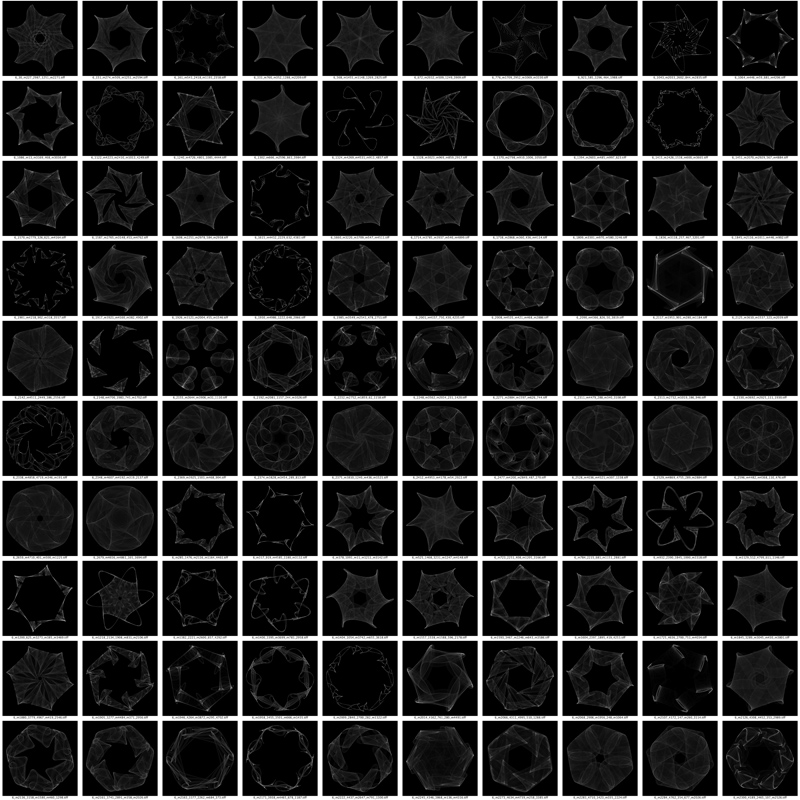6 fold symmetry

Cases with both mirror and orientation symmetry, omega=0.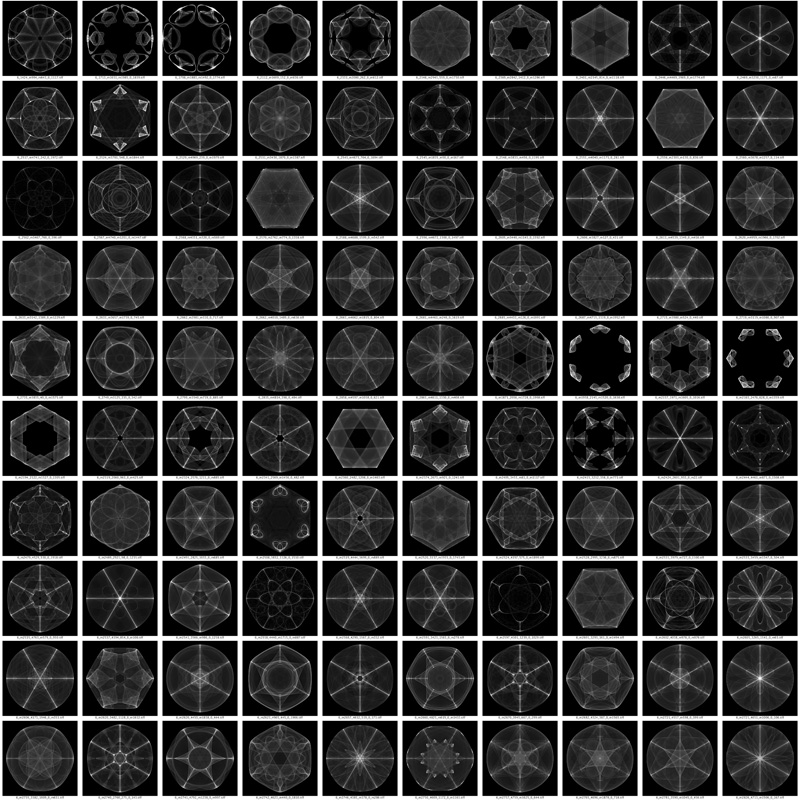6 fold symmetry3 fold symmetry

8 and 12 fold symmetry tables.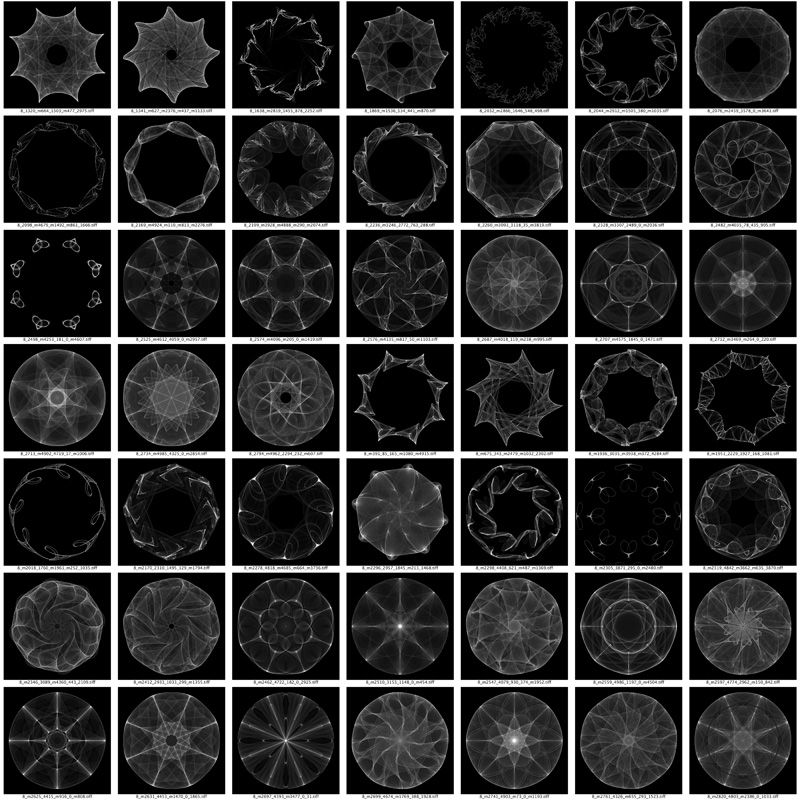8 fold symmetry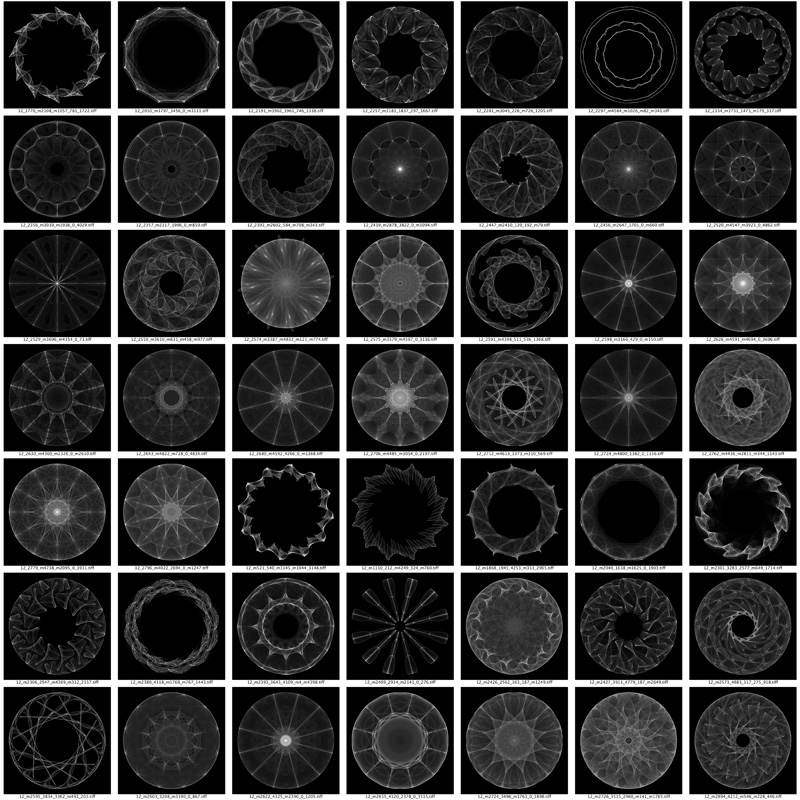12 fold symmetry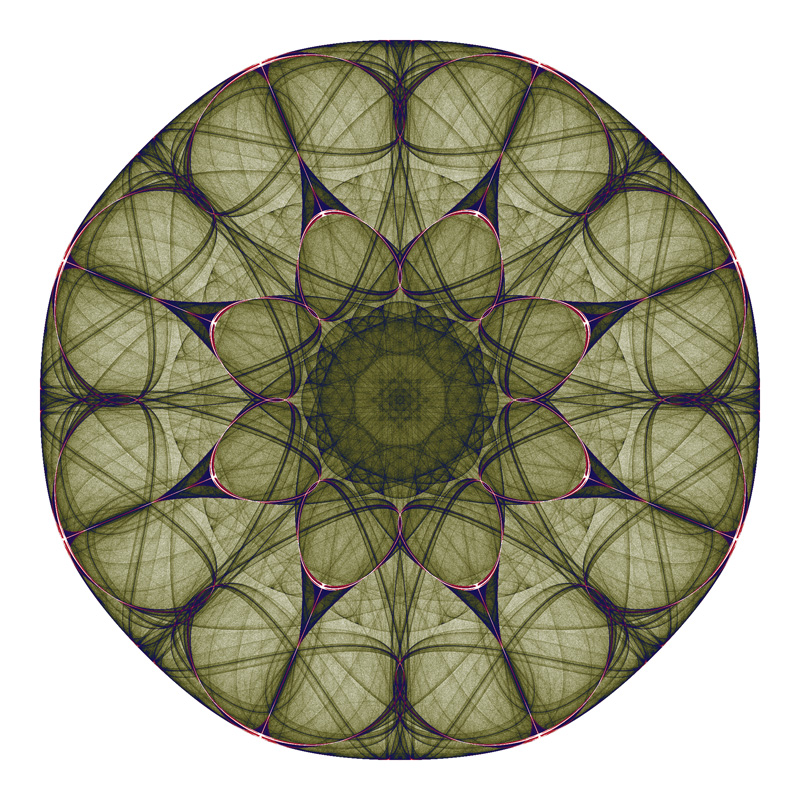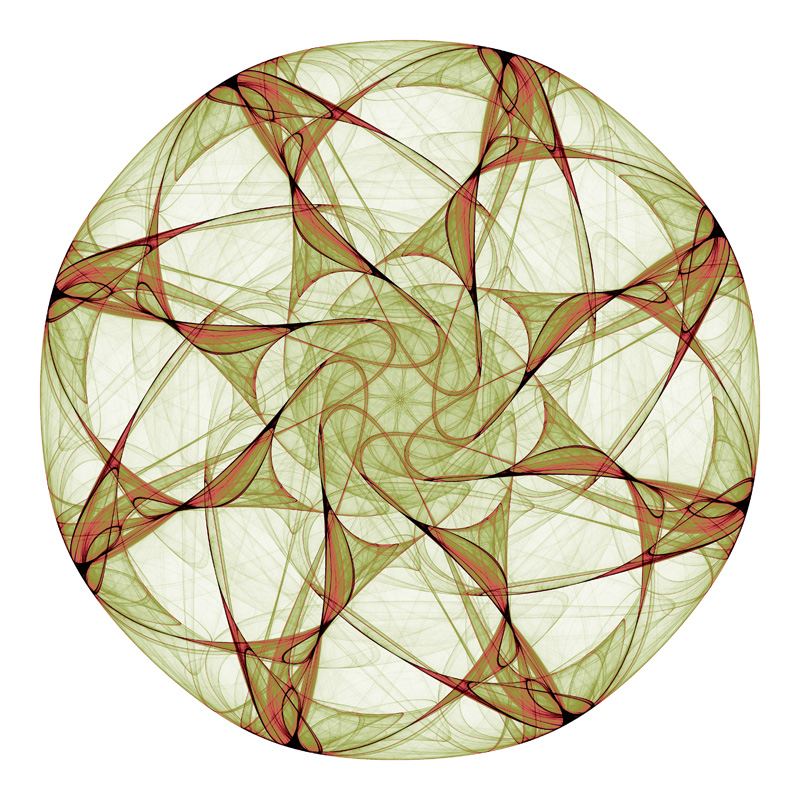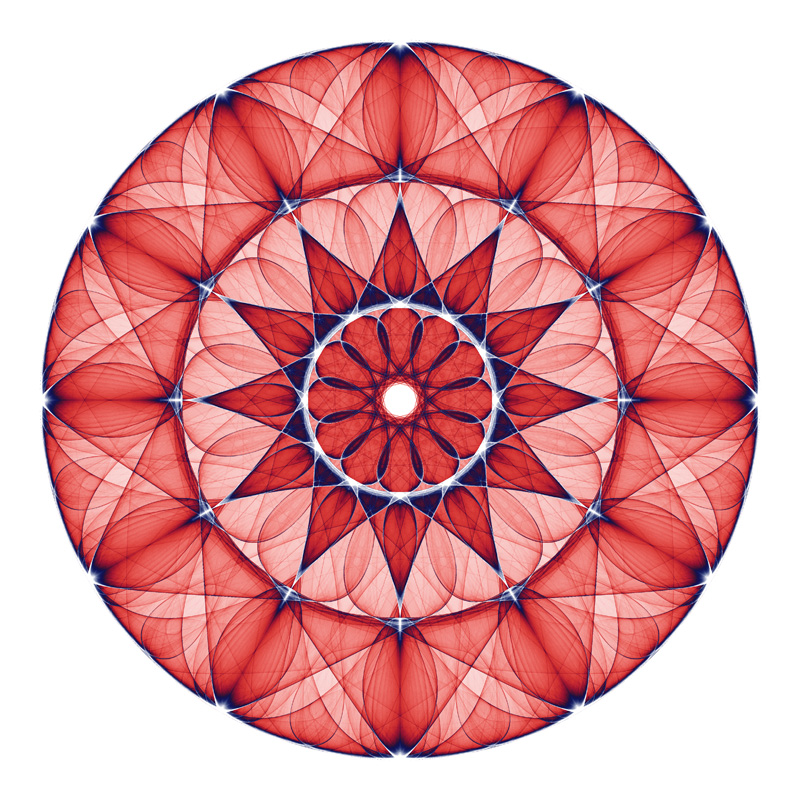References

• Symmetric Chaos: How and Why. Notices of the American Mathematical Society · January 1995. Mike Field and Martin Golubitsky.

• Symmetric Chaos. Computers in Physics 4, 470 (1990); doi: 10.1063/1.4822939. Mike Field and Martin Golubitsky.

• Singularities and Groups in Bifurcation Theory. Singularities and Groups in Bifurcation Theory: Vol. lI. Appl. Math. Sci. 69, Springer-V erlag, New Y ork, 1988. M. Golubitsky, I. N. Stewart and D. G. Schaeffer.

• Symmetry groups of attractors. Arch. Rational Mech. & Anal. 126 (1994), 59–78. P. Ashwin and I. Melbourne.

• Symmetry increasing bifurcation of chaotic attractors. Physica D 32 (1988), 423–436. P. Chossat and M. Golubitsky.

• A note on symmetries of invariant sets with compact group actions. In Equadiff 8, Tatra Mountains Math. Publ. 4 (1994), 93–104. M. Field, M. Golubitsky, and M. Nicol.

• Symmetric Chaos. In Nonlinear Equations in the Applied Sciences, (W.F. Ames & C.F. Rogers, eds.), Academic Press, 1991, 257–315. G. King and I. Stewart.

• The structure of symmetric attractors. Arch. Rational Mech. & Anal. 123 (1993), 75–98. I. Melbourne, M. Dellnitz, and M. Golubitsky

• Fearful symmetry: Is god a geometer? Blackwell Publishers, Oxford, 1992. I. Stewart and M. Golubitsky.